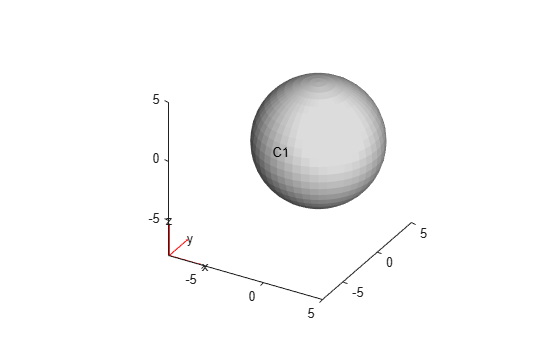# Cuboids, Cylinders, and Spheres

This example shows how to create 3-D geometries formed by one or more cubic, cylindrical, and spherical cells by using the `multicuboid`, `multicylinder`, and `multisphere` functions, respectively. With these functions, you can create stacked or nested geometries. You also can create geometries where some cells are empty; for example, hollow cylinders, cubes, or spheres.

All cells in a geometry must be of the same type: either cuboids, or cylinders, or spheres. These functions do not combine cells of different types in one geometry.

### Single Sphere

Create a geometry that consists of a single sphere and include this geometry in a PDE model.

Use the `multisphere` function to create a single sphere. The resulting geometry consists of one cell.

`gm = multisphere(5)`
```gm = DiscreteGeometry with properties: NumCells: 1 NumFaces: 1 NumEdges: 0 NumVertices: 0 Vertices: [] ```

Create a PDE model.

`model = createpde`
```model = PDEModel with properties: PDESystemSize: 1 IsTimeDependent: 0 Geometry: [] EquationCoefficients: [] BoundaryConditions: [] InitialConditions: [] Mesh: [] SolverOptions: [1x1 pde.PDESolverOptions] ```

Include the geometry in the model.

`model.Geometry = gm`
```model = PDEModel with properties: PDESystemSize: 1 IsTimeDependent: 0 Geometry: [1x1 DiscreteGeometry] EquationCoefficients: [] BoundaryConditions: [] InitialConditions: [] Mesh: [] SolverOptions: [1x1 pde.PDESolverOptions] ```

Plot the geometry.

`pdegplot(model,'CellLabels','on')`### Nested Cuboids of Same Height

Create a geometry that consists of three nested cuboids of the same height and include this geometry in a PDE model.

Create the geometry by using the `multicuboid` function. The resulting geometry consists of three cells.

`gm = multicuboid([2 3 5],[4 6 10],3)`
```gm = DiscreteGeometry with properties: NumCells: 3 NumFaces: 18 NumEdges: 36 NumVertices: 24 Vertices: [24x3 double] ```

Create a PDE model.

`model = createpde`
```model = PDEModel with properties: PDESystemSize: 1 IsTimeDependent: 0 Geometry: [] EquationCoefficients: [] BoundaryConditions: [] InitialConditions: [] Mesh: [] SolverOptions: [1x1 pde.PDESolverOptions] ```

Include the geometry in the model.

`model.Geometry = gm`
```model = PDEModel with properties: PDESystemSize: 1 IsTimeDependent: 0 Geometry: [1x1 DiscreteGeometry] EquationCoefficients: [] BoundaryConditions: [] InitialConditions: [] Mesh: [] SolverOptions: [1x1 pde.PDESolverOptions] ```

Plot the geometry.

`pdegplot(model,'CellLabels','on','FaceAlpha',0.5)`### Stacked Cylinders

Create a geometry that consists of three stacked cylinders and include this geometry in a PDE model.

Create the geometry by using the `multicylinder` function with the `ZOffset` argument. The resulting geometry consists of four cells stacked on top of each other.

`gm = multicylinder(10,[1 2 3 4],'ZOffset',[0 1 3 6])`
```gm = DiscreteGeometry with properties: NumCells: 4 NumFaces: 9 NumEdges: 5 NumVertices: 5 Vertices: [5x3 double] ```

Create a PDE model.

`model = createpde`
```model = PDEModel with properties: PDESystemSize: 1 IsTimeDependent: 0 Geometry: [] EquationCoefficients: [] BoundaryConditions: [] InitialConditions: [] Mesh: [] SolverOptions: [1x1 pde.PDESolverOptions] ```

Include the geometry in the model.

`model.Geometry = gm`
```model = PDEModel with properties: PDESystemSize: 1 IsTimeDependent: 0 Geometry: [1x1 DiscreteGeometry] EquationCoefficients: [] BoundaryConditions: [] InitialConditions: [] Mesh: [] SolverOptions: [1x1 pde.PDESolverOptions] ```

Plot the geometry.

`pdegplot(model,'CellLabels','on','FaceAlpha',0.5)`### Hollow Cylinder

Create a hollow cylinder and include it as a geometry in a PDE model.

Create a hollow cylinder by using the `multicylinder` function with the `Void` argument. The resulting geometry consists of one cell.

`gm = multicylinder([9 10],10,'Void',[true,false])`
```gm = DiscreteGeometry with properties: NumCells: 1 NumFaces: 4 NumEdges: 4 NumVertices: 4 Vertices: [4x3 double] ```

Create a PDE model.

`model = createpde`
```model = PDEModel with properties: PDESystemSize: 1 IsTimeDependent: 0 Geometry: [] EquationCoefficients: [] BoundaryConditions: [] InitialConditions: [] Mesh: [] SolverOptions: [1x1 pde.PDESolverOptions] ```

Include the geometry in the model.

`model.Geometry = gm`
```model = PDEModel with properties: PDESystemSize: 1 IsTimeDependent: 0 Geometry: [1x1 DiscreteGeometry] EquationCoefficients: [] BoundaryConditions: [] InitialConditions: [] Mesh: [] SolverOptions: [1x1 pde.PDESolverOptions] ```

Plot the geometry.

`pdegplot(model,'CellLabels','on','FaceAlpha',0.5)`